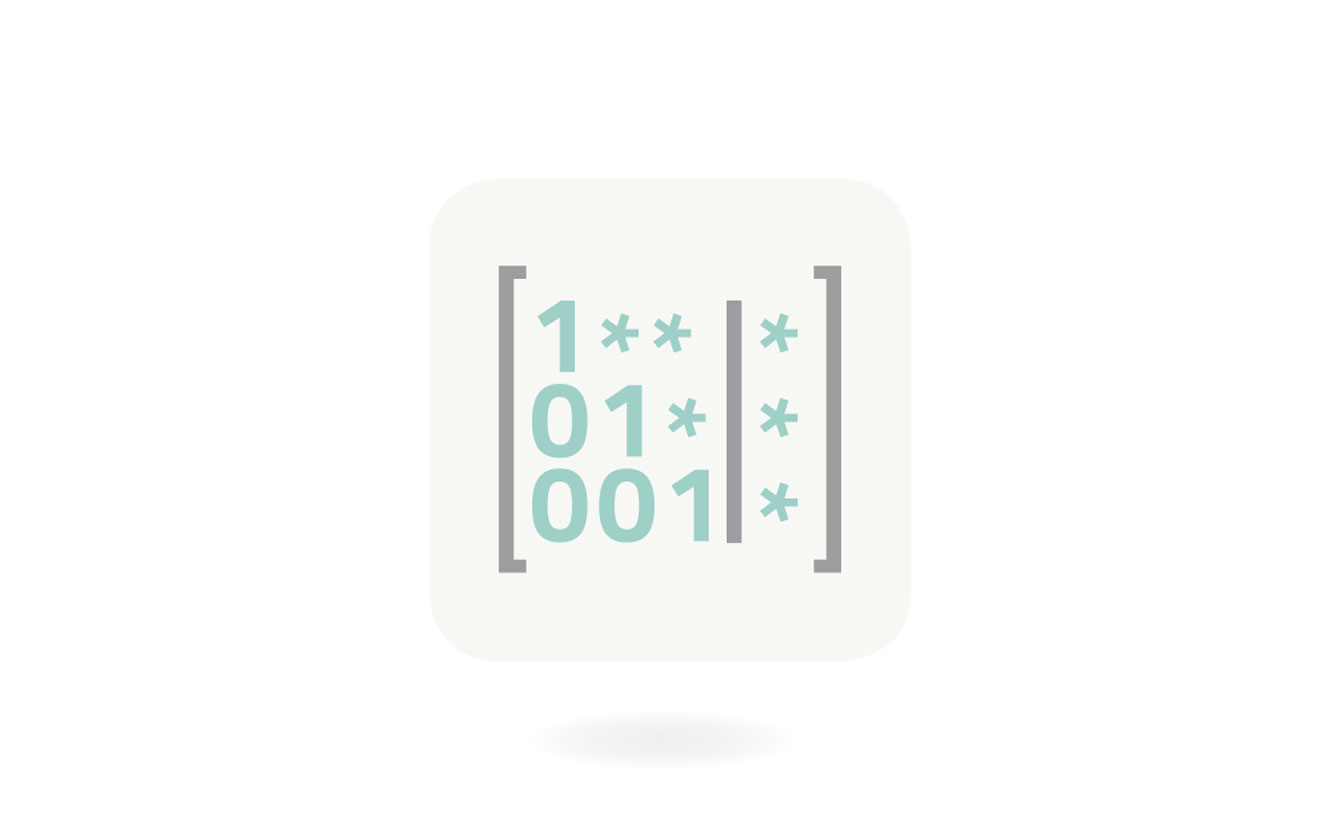# Linear Algebra

## Self-Paced Hands-On Linear Algebra Course

Linear Algebra is an essential skill set required in Data Science, engineering, and many other areas. In this 80-hour hands-on college-level course students learn by doing, at their own pace, completing hands-on interactive coursework with either in-person or remote support of a dedicated personal tutor.## What You Will Learn

Linear Algebra studies the solution of (possibly very large) systems of linear algebraic equations. It is an integral part of many scientific and engineering applications including page ranking in search engines, machine learning, artificial intelligence, predictive data analytics, optimization and linear programming, data encoding, signal analysis, computer graphics, image processing and face recognition, quantum computing, engineering calculations (such as deformations or electromagnetics fields, …), and many others.

But why should we be the ones explaining the importance of Linear Algebra when there is Stack Exchange? Here is a sample answer to “Why study Linear Algebra?”:

“Having studied Engineering, I can tell you that Linear Algebra is fundamental and an extremely powerful tool in every single discipline of Engineering. If you are reading this and considering learning Linear Algebra then I will first issue you with a warning: Linear Algebra is mighty stuff. You should be both maniacally excited and scared by the awesome power it will give you!!!!!!”      user1158559

## Recommended Background

To succeed in this course, you should know basic high school math including arithmetic, working with fractions, relations, and functions. You should also know trigonometric functions, exponentials, and logarithms. Later we will work with inner products of polynomials which requires a basic knowledge of integration.

## Student Learning Outcomes (SLO)

Students will be able to:

• Perform all standard vector and matrix operations.
• Transform linear system to matrix form and solve them.
• Create echelon and reduced echelon forms of matrices.
• Determine basic and free variables in linear systems.
• Express infinite solution sets in parametric vector form.
• Work with determinants and elementary matrices.
• Create and use LU and Cholesky matrix factorizations.
• Work with linear transformations associated with matrices.
• Calculate eigenvalues and eigenvectors.
• Work with linear spaces, bases, and coordinates.
• Perform orthogonal projections and decompositions.
• Diagonalize matrices.
• Perform spectral decomposition of matrices.
• Solve Least-Squares problems via normal equations and QR factorization.
• Perform Singular Value Decomposition (SVD) of matrices.
• Apply SVD to data cleaning and image compression.

## Equipment Requirements

Computer, laptop or tablet with Internet access, email, and one of the following browsers: